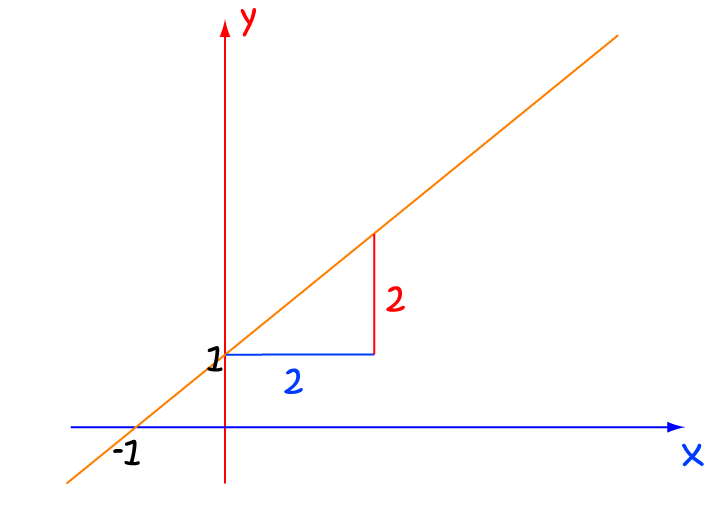Pull Down & Release to Refresh

# Math Definitions - Letter G

### ChaptersThe gradient tells you how steep a straight line is.

The gradient is equal to $\dfrac{\text{vertical rise}}{\text{horizontal run}}$.

The gradient of the line in the picture is $\dfrac{\text{vertical rise}}{\text{horizontal run}} = \dfrac{2}{2} = 1$.

Another name for the gradient is the slope.

### Description

The aim of this dictionary is to provide definitions to common mathematical terms. Students learn a new math skill every week at school, sometimes just before they start a new skill, if they want to look at what a specific term means, this is where this dictionary will become handy and a go-to guide for a student.

### Audience

Year 1 to Year 12 students

### Learning Objectives

Learn common math terms starting with letter G

Author: Subject Coach
You must be logged in as Student to ask a Question.